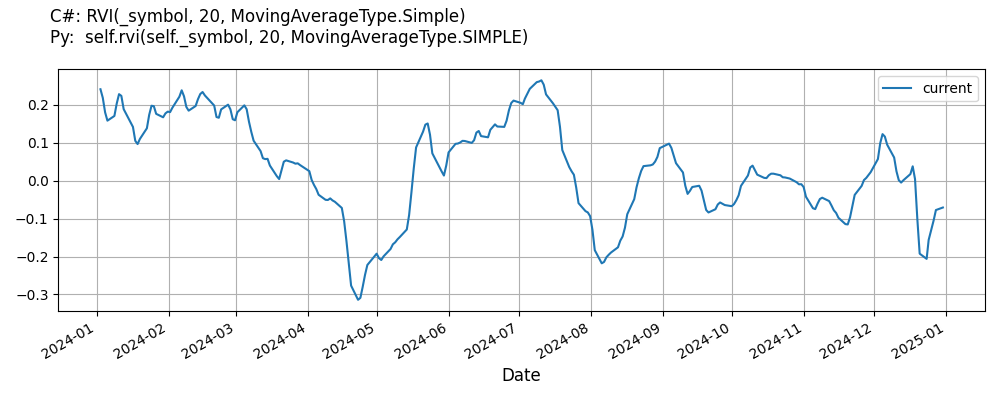# Supported Indicators

## Relative Vigor Index

### Introduction

The Relative Vigor Index (RVI) compares the ratio of the closing price of a security to its trading range. For illustration, let: a = Close−Openb = Close−Open of One Bar Prior to ac = Close−Open of One Bar Prior to bd = Close−Open of One Bar Prior to ce = High−Low of Bar af = High−Low of Bar bg = High−Low of Bar ch = High−Low of Bar d Then let (a+2*(b+c)+d)/6 be NUM and (e+2*(f+g)+h)/6 be DENOM. RVI = SMA(NUM)/SMA(DENOM) for a specified period. https://www.investopedia.com/terms/r/relative_vigor_index.asp

To view the implementation of this indicator, see the LEAN GitHub repository.

### Using RVI Indicator

To create an automatic indicators for RelativeVigorIndex, call the RVI helper method from the QCAlgorithm class. The RVI method creates a RelativeVigorIndex object, hooks it up for automatic updates, and returns it so you can used it in your algorithm. In most cases, you should call the helper method in the Initialize method.

public class RelativeVigorIndexAlgorithm : QCAlgorithm
{
private Symbol _symbol;
private RelativeVigorIndex _rvi;

public override void Initialize()
{
_rvi = RVI(_symbol, 20, MovingAverageType.Simple);
}

public override void OnData(Slice data)
{
{
// The current value of _rvi is represented by itself (_rvi)
// or _rvi.Current.Value
Plot("RelativeVigorIndex", "rvi", _rvi);
// Plot all properties of rvi
Plot("RelativeVigorIndex", "signal", _rvi.Signal);
}
}
}
class RelativeVigorIndexAlgorithm(QCAlgorithm):
def Initialize(self) -> None:
self.rvi = self.RVI(self.symbol, 20, MovingAverageType.Simple)

def OnData(self, slice: Slice) -> None:
# The current value of self.rvi is represented by self.rvi.Current.Value
self.Plot("RelativeVigorIndex", "rvi", self.rvi.Current.Value)
# Plot all attributes of self.rvi
self.Plot("RelativeVigorIndex", "signal", self.rvi.Signal.Current.Value)


The following reference table describes the RVI method:

### RVI()1/1

            RelativeVigorIndex QuantConnect.Algorithm.QCAlgorithm.RVI (
Symbol                            symbol,
Int32                             period,
*MovingAverageType                movingAverageType,
*Nullable<Resolution>       resolution,
*Func<IBaseData, TradeBar>  selector
)


Creates a new RelativeVigorIndex indicator.

If you don't provide a resolution, it defaults to the security resolution. If you provide a resolution, it must be greater than or equal to the resolution of the security. For instance, if you subscribe to hourly data for a security, you should update its indicator with data that spans 1 hour or longer.

The following table describes the MovingAverageType enumeration members:

You can manually create a RelativeVigorIndex indicator, so it doesn't automatically update. Manual indicators let you update their values with any data you choose.

Updating your indicator manually enables you to control when the indicator is updated and what data you use to update it. To manually update the indicator, call the Update method with a TradeBar, or QuoteBar. The indicator will only be ready after you prime it with enough data.

public class RelativeVigorIndexAlgorithm : QCAlgorithm
{
private Symbol _symbol;
private RelativeVigorIndex _rvi;

public override void Initialize()
{
_rvi = new RelativeVigorIndex(20, MovingAverageType.Simple);
}

public override void OnData(Slice data)
{
if (data.Bars.TryGeValue(_symbol, out var bar))
{
_rvi.Update(bar);
}

{
// The current value of _rvi is represented by itself (_rvi)
// or _rvi.Current.Value
Plot("RelativeVigorIndex", "rvi", _rvi);
// Plot all properties of rvi
Plot("RelativeVigorIndex", "signal", _rvi.Signal);
}
}
}
class RelativeVigorIndexAlgorithm(QCAlgorithm):
def Initialize(self) -> None:
self.rvi = RelativeVigorIndex(20, MovingAverageType.Simple)

def OnData(self, slice: Slice) -> None:
bar = slice.Bars.get(self.symbol)
if bar:
self.rvi.Update(bar)

# The current value of self.rvi is represented by self.rvi.Current.Value
self.Plot("RelativeVigorIndex", "rvi", self.rvi.Current.Value)
# Plot all attributes of self.rvi
self.Plot("RelativeVigorIndex", "signal", self.rvi.Signal.Current.Value)


To register a manual indicator for automatic updates with the security data, call the RegisterIndicator method.

public class RelativeVigorIndexAlgorithm : QCAlgorithm
{
private Symbol _symbol;
private RelativeVigorIndex _rvi;

public override void Initialize()
{
_rvi = new RelativeVigorIndex(20, MovingAverageType.Simple);
RegisterIndicator(_symbol, _rvi, Resolution.Daily);
}

public override void OnData(Slice data)
{
{
// The current value of _rvi is represented by itself (_rvi)
// or _rvi.Current.Value
Plot("RelativeVigorIndex", "rvi", _rvi);
// Plot all properties of rvi
Plot("RelativeVigorIndex", "signal", _rvi.Signal);
}
}
}
class RelativeVigorIndexAlgorithm(QCAlgorithm):
def Initialize(self) -> None:
self.rvi = RelativeVigorIndex(20, MovingAverageType.Simple)
self.RegisterIndicator(self.symbol, self.rvi, Resolution.Daily)

def OnData(self, slice: Slice) -> None:
# The current value of self.rvi is represented by self.rvi.Current.Value
self.Plot("RelativeVigorIndex", "rvi", self.rvi.Current.Value)
# Plot all attributes of self.rvi
self.Plot("RelativeVigorIndex", "signal", self.rvi.Signal.Current.Value)


The following reference table describes the RelativeVigorIndex constructor:

### RelativeVigorIndex()1/2

            RelativeVigorIndex QuantConnect.Indicators.RelativeVigorIndex (
int                period,
MovingAverageType  type
)


Initializes a new instance of the RelativeVigorIndex (RVI) class.

### RelativeVigorIndex()2/2

            RelativeVigorIndex QuantConnect.Indicators.RelativeVigorIndex (
string              name,
int                 period,
*MovingAverageType  type
)


Initializes a new instance of the RelativeVigorIndex (RVI) class.

### Visualization

The following image shows plot values of selected properties of RelativeVigorIndex using the plotly library.You can also see our Videos. You can also get in touch with us via Discord.